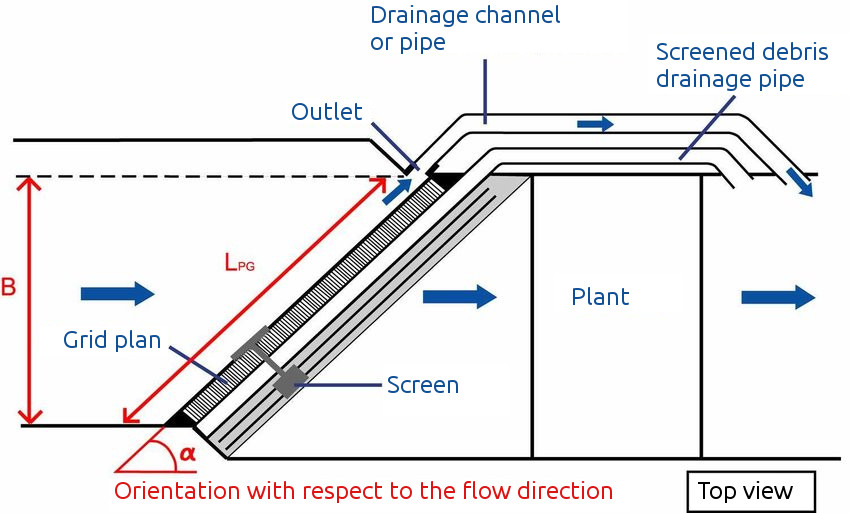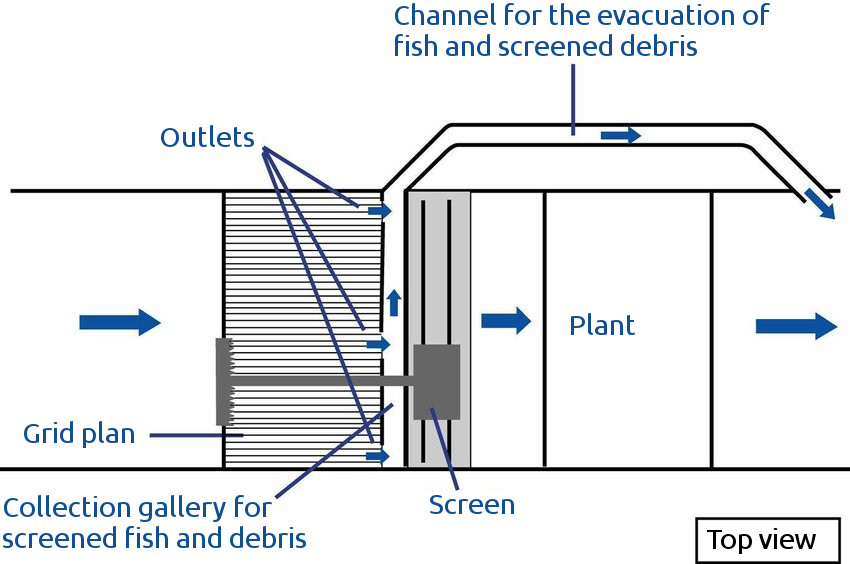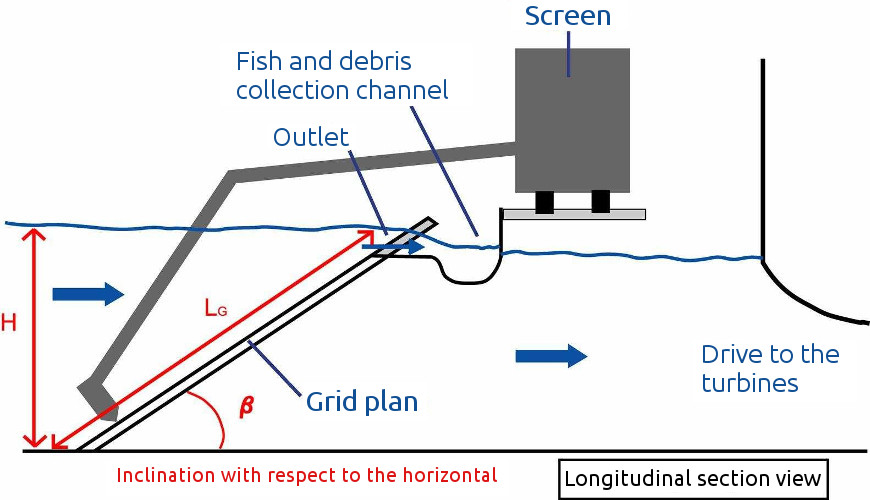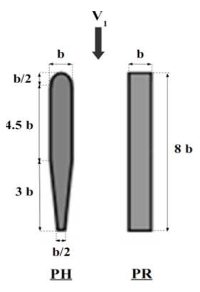# Calculation of the head loss on a water intake trashrack

Head loss $$\Delta H$$ on a water intake trashrack is calculated as follows:

$\Delta H = \xi \frac{V_1^2}{2g}$

Upstream flow velocity $$V_1$$ is calculated from the discharge $$Q$$, the water height $$H_1$$ and the intake width $$B$$ upstream the trashrack:

$V_1 = \frac{Q}{H_1 \times B}$

The calculation of the head loss coefficient $$\xi$$ is based on the characteristics of the trashrack. For a full description of the assumptions, formulas and limitations of the method, please refer to the Raynal et al. (2012) report1 available here (in French): https://continuite-ecologique.fr/wp-content/uploads/2019/11/2012_014.pdf

## Conventional trashrack

Conventional trashracks: perpendicular to the flow and slightly inclined to the horizontal.

### Formula

Use of the F1 formula of Raynal et al (2012)1 to calculate the head losses.

$\xi = a * K_O * K_\beta$

With:

• $$\xi$$: total head loss coefficient (-)
• $$a$$: bar shape coefficient (-), see "Bar profile" below
• $$K_O$$: blockage head loss coefficient due to bars, spacers and clogging (-)
• $$K_\beta$$: head loss coefficient due to the angle of the trashrack (-)
$K_O = \left ( \frac{O_C}{1-O_C} \right )^{1.6}$

With $$O_C$$ blockage ratio due to bars, spacers and clogging (-):

$O_C = O + (1 - O) \times C$

With:

• $$O$$ blockage ratio due to bars and spacers
• $$C$$ clogging ratio
$K_\beta = \left ( 1 - \cos{\beta} \right )^{0.39}$

With $$\beta$$ the angle of inclination of the trashrack in reference to the vertical (°).

## Angled trashrack

Flow-oriented and near-vertical trashracks.Courret, D. et Larinier, M. Guide pour la conception de prise d’eau ichtyocompatibles pour les petites centrales hydroélectriques, 2008. https://doi.org/10.13140/RG.2.1.2359.1449.

### Formula

Use of the F2 formula of Raynal et al (2012)1 to calculate the head losses (Also in Equation (7) of Raynal et al. (2013)2).

$\xi = a * K_O * K_\alpha$

With $$K_\alpha$$ head loss coefficient due to inclination in reference to the horizontal (-)

$K_\alpha = 1 + c * \left ( \frac{90 - \alpha}{90} \right )^{2.35} * \left ( \frac{1 - O}{O} \right )^{3}$

With:

• $$\alpha$$: inclination angle of the trashrack in reference to the horizontal (°)
• $$c$$: bar shape coefficient (-), see "Bar profile" below

## Inclined trashrack

trashracks perpendicular to the flow, and inclined with respect to the horizontalCourret, D. et Larinier, M. Guide pour la conception de prise d’eau ichtyocompatibles pour les petites centrales hydroélectriques, 2008. https://doi.org/10.13140/RG.2.1.2359.1449.

### Formula

Use of the F3 formula of Raynal et al (2012)1 to calculate the head losses (Also in Equation (11) of Raynal et al. (2013)3).

$\xi = a * K_b * K_\beta + K_{Fent} * K_{entH}$

With:

• $$K_b$$: blockage head loss coefficient due to bars and clogging (-)
• $$K_{Fent}$$: spacer shape coefficient (-), see "Bar profile" below
• $$K_{entH}$$: blockage head loss coefficient due to spacers (-)
$K_b = \left ( \frac{O_{b,C}}{1-O_{b,C}} \right )^{1.65}$

With $$O_{b,C} = O_b + (1 - O_b) \times C$$ et $$O_b$$ blockage ratio due to bars.

$K_\beta = \left ( \sin \beta \right )^{2}$
$K_{entH} = \left ( \frac{O_{entH}}{1-O_{entH}} \right )^{0.77}$

With $$O_{entH}$$ blockage ratio due to spacers.

## Parameters

### Elevation of the immersed vertex of the trashrack

May be different from the water level if the top of the trashrack is drowned.

### Section width $$B$$

For conventional or inclined trashrack, it mMust also correspond to the width of the trashrack.

### Average approach speed for the maximum turbinated flow, subtracting the upper blocked part, if any

"Maximum" value of the approach speed taken into account in the calculation of the head loss in a safety approach.

### Inclination with respect to the horizontal

#### Conventional trashrack

Scope of the formula: 45 ≤ β ≤ 90°

#### Angled trashrack

Vertical trashracks (β = 90°).

The slight inclination of the trashracks (β≈ 75/80°), often set up for screening purposes, can be neglected.

#### inclined trashrack

Scope of the formula: 15° ≤ β ≤ 90°

Recommended for fish guidance: β ≤ 26°

### Orientation with respect to the direction of flow

#### Conventional trashrack

trashracks perpendicular to the flow (α = 90°)

#### Angled trashrack

Scope of the formula: 30° ≤ α ≤ 90°

Recommended for fish guidance: α ≤ 45°

#### inclined trashrack

trashracks perpendicular to the flow (α = 90°)

### Average normal speed for maximum turbinated flow rate $$V_N$$

Recommended to avoid plating fish on the trashrack (physical barrier) or their premature passage through (behavioural barrier): VN ≤ 0.5 m/s.

#### Angled or inclined trashrack

Above the average value calculated here, it is essential to refer to the recommendations derived from the experimental characterization of the actual velocity values.

### Bar shape ratio $$b/p$$

#### Angled trashrack

Validity range of the formula: ratio $$b/p$$ close to 0.125

### Ratio of spacing / bar thickness

#### Angled trashrack

Scope of validity of the formula: $$1 \leq e / b \leq 3$$ with $$e$$ space between bars.

### Overall obstruction for conventional and angled trashracks

Calculated from bars + spacers + longitudinal and transverse support elements retained, it has to be determined from the trashrack plans.

Scope of validity of the formula for conventional trashracks: $$0.2 \leq O \leq 0.60$$

Scope of validity of the formula for angled trashracks: $$0.35 \leq O \leq 0.60$$

### Overall obstruction for inclined trashracks

It consists of two parts.

Obstruction due to the bars and longitudinal support elements retained $$O_b$$. To be determined from the trashrack plans.. It must be higher or equal to bar blockage ratio given by $$b / (b + e)$$. Scope of validity of the formula: $$0.28 \leq O \leq 0.53$$.

Blockage ratio due to spacers. Scope of validity of the formula: $$O_{entH} \leq 0.28$$

### Bar profileRaynal, Sylvain. « Étude expérimentale et numérique des grilles ichtyocompatibles ». Sciences et ingénierie en matériaux, mécanique, énergétique et aéronautique - SIMMEA, 2013.

#### Conventional trashrack

The shape coefficient of the bars $$a$$ is 2.89 for the rectangular profile (PR) and 1.70 for the hydrodynamic profile (PH).

#### Angled trashrack

The shape coefficient of the bars is 2.89 for the rectangular profile (PR) and 1.70 for the hydrodynamic profile (PH).

The shape coefficient of the bars $$c$$ is 1.69 for the rectangular profile (PR) and 2.78 for the hydrodynamic profile (PH).

#### inclined trashrack

Bar coefficient $$A_i$$ 2.47 1.75 1.27 1.79 2.10 3.85

After Lemkecher et al. (2020)4

### Average shape coefficient of spacers and transverse elements, weighted according to their respective shares

#### inclined trashrack

To be determined from the trashrack plans.

For example, 1.79 for cylindrical spacers, 2.42 for rectangular spacers, and around 4 for square beams and IPNs.

1. Raynal, S., Courret, D., Chatellier, L., Larinier, M., David, L., 2012. Définition de prises d’eau ichtyocompatibles -Pertes de charge au passage des plans de grille inclinés ou orientés dans des configurations ichtyocompatibles et champs de vitesse à leur approche (POLE RA11.02). https://continuite-ecologique.fr/wp-content/uploads/2019/11/2012_014.pdf

2. Raynal, S., Chatellier, L., Courret, D., Larinier, M., David, L., 2013. An experimental study on fish-friendly trashracks–Part 2. Angled trashracks. Journal of Hydraulic Research 51, 67–75. https://doi.org/10.1080/00221686.2012.753646

3. Raynal, S., Courret, D., Chatellier, L., Larinier, M., David, L., 2013. An experimental study on fish-friendly trashracks–Part 1. Inclined trashracks. Journal of Hydraulic Research 51, 56–66. https://doi.org/10.1080/00221686.2012.753647

4. Lemkecher, F., Chatellier, L., Courret, D., David, L., 2020. Contribution of Different Elements of Inclined Trash Racks to Head Losses Modeling. Water 12, 966. https://doi.org/10.3390/w12040966